Courses

# NEET Previous Year Questions (2014-20): Nuclei Notes | EduRev

## Class 12 : NEET Previous Year Questions (2014-20): Nuclei Notes | EduRev

The document NEET Previous Year Questions (2014-20): Nuclei Notes | EduRev is a part of the Class 12 Course Physics Class 12.
All you need of Class 12 at this link: Class 12

Q.1. The energy equivalent of 0.5 g of a substance is :     (2020)
A: 1.5×1013 J
B: 0.5×1013J
C: 4.5×1016J
D: 4.5×1013J

Ans: D
Solution:
E = Δmc2 = 0.5 × 10-3 × (3 × 108)2
= 0.5 ×10-3 × 9 ×1016
E = 4.5 ×1013 J

Q.2. When a uranium isotope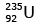is bombarded with a neutron, it generates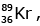three neutrons and :      (2020)
A: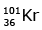B: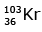C: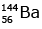D: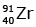Ans: C
Solution: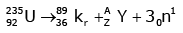92 = 36 + Z; ← A + 89 + 3 = 235 ⇒ A = 144
Z = 56

Q.3. For a radioactive material, half-life is 10 minutes. If initially there are 600 number of nuclei, the time taken (in minutes) for the disintegration of 450 nuclei is:    (2018)
A: 20
B: 10
C: 30
D: 15
Ans:
A
Solution: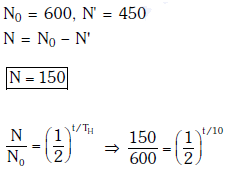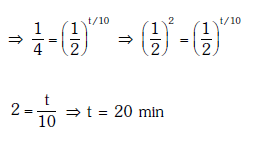Q.4. Radioactive material 'A' has decay constant '8 λ' and material 'B' has decay constant 'λ'. Initially they have same number of nuclei. After what time, the ratio of number of nuclei of material 'B' to that 'A' will be 1/e?     (2017)
A: 1/7λ
B: 1/8λ
C: 1/9λ
D: 1/10λ
Ans: A
Solution: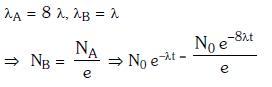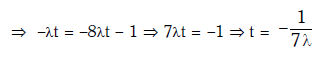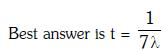Q.5. The Binding energy per nucleon of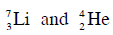nuclei are 5.60 MeV and 7.06 MeV, respectively. In the nuclear reaction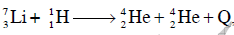the value of energy Q released is :    (2014)
A: 8.4 MeV
B: 17.3 MeV
C: 19.6 MeV
D: −2.4 MeV
Ans: B
Solution: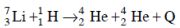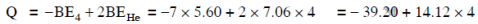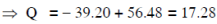Q.6. A radio isotope ‘X’ with a half life 1.4 × 109 years decays to ‘Y’ which is stable. A sample of the rock from a cave was found to contain ‘X’ and ‘Y’ in the ratio 1 : 7. The age of the rock is    (2014)
A: 4.20 × 109 years
B: 8.40 × 109 years
C: 1.96 × 109 years
D: 3.92 × 109 years
Ans: A
Solution:

Ratio of X:Y is given = 1:7
That is,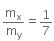⇒ 7mx = my
Let, the initial total mass is m.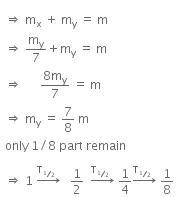Therefore, time taken to become 1/8 unstable part
= 3 x T1/2
= 3 x 1.4 x 109
= 4.2 x 109 y

Offer running on EduRev: Apply code STAYHOME200 to get INR 200 off on our premium plan EduRev Infinity!

## Physics Class 12

132 videos|306 docs|130 tests

,

,

,

,

,

,

,

,

,

,

,

,

,

,

,

,

,

,

,

,

,

;